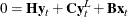The SIMLIN Procedure

EST= Data Set

Normally, PROC SIMLIN uses an EST= data set produced by PROC SYSLIN with the OUTEST= option. This data set is in the form expected by PROC SIMLIN. If there is more than one set of estimates produced by PROC SYSLIN, you must use the TYPE= option in the PROC SIMLIN statement to select the set to be simulated. Then PROC SIMLIN reads from the EST= data set only those observations with a _TYPE_ value corresponding to the TYPE= option (for example, TYPE=2SLS) or with a _TYPE_ value of IDENTITY.

The SIMLIN procedure can only solve square, nonsingular systems. If you have fewer equations than endogenous variables, you must specify IDENTITY statements in the PROC SYSLIN step to bring the system up to full rank. If there are g endogenous variables and m <g stochastic equations with unknown parameters, then you use m MODEL statements to specify the equations with parameters to be estimated and you must use g-m IDENTITY statements to complete the system.

You can build your own EST= data set with a DATA step rather than use PROC SYSLIN. The EST= data set must contain the endogenous variables, the lagged endogenous variables (if any), and the exogenous variables in the system (if any). If any of the equations have intercept terms, the variable INTERCEPT must supply these coefficients. The EST= data set should also contain the special character variable comp _DEPVAR_ to label the equations.

The EST= data set must contain one observation for each equation in the system. The values of the lagged endogenous variables must contain the C coefficients. The values of the exogenous variables and the INTERCEPT variable must contain the B coefficients. The values of the endogenous variables, however, must contain the negatives of the G coefficients. This is because the SYSLIN procedure writes the coefficients to the OUTEST= data set in the formwhere H =-G.

See "Multipliers for Higher Order Lags" and Example 25.2 later in this chapter for more information on building the EST= data set.